# 约定

``````public abstract class Sort<T extends Comparable<T>> {

public abstract void sort(T[] nums);

protected boolean less(T v, T w) {
return v.compareTo(w) < 0;
}

protected void swap(T[] a, int i, int j) {
T t = a[i];
a[i] = a[j];
a[j] = t;
}
}
``````

# 选择排序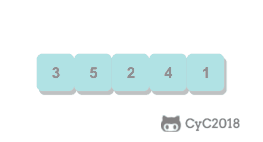``````public class Selection<T extends Comparable<T>> extends Sort<T> {

@Override
public void sort(T[] nums) {
int N = nums.length;
for (int i = 0; i < N - 1; i++) {
int min = i;
for (int j = i + 1; j < N; j++) {
if (less(nums[j], nums[min])) {
min = j;
}
}
swap(nums, i, min);
}
}
}
``````

# 冒泡排序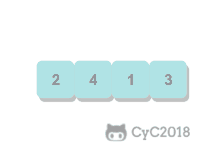``````public class Bubble<T extends Comparable<T>> extends Sort<T> {

@Override
public void sort(T[] nums) {
int N = nums.length;
boolean isSorted = false;
for (int i = N - 1; i > 0 && !isSorted; i--) {
isSorted = true;
for (int j = 0; j < i; j++) {
if (less(nums[j + 1], nums[j])) {
isSorted = false;
swap(nums, j, j + 1);
}
}
}
}
}
``````

# 插入排序

• 平均情况下插入排序需要 \~N2/4 比较以及 \~N2/4 次交换；
• 最坏的情况下需要 \~N2/2 比较以及 \~N2/2 次交换，最坏的情况是数组是倒序的；
• 最好的情况下需要 N-1 次比较和 0 次交换，最好的情况就是数组已经有序了。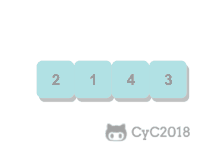``````public class Insertion<T extends Comparable<T>> extends Sort<T> {

@Override
public void sort(T[] nums) {
int N = nums.length;
for (int i = 1; i < N; i++) {
for (int j = i; j > 0 && less(nums[j], nums[j - 1]); j--) {
swap(nums, j, j - 1);
}
}
}
}
``````

# 希尔排序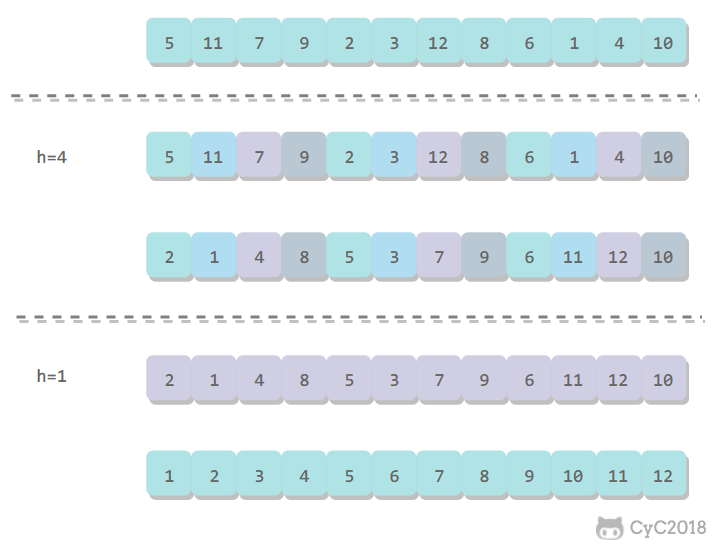``````public class Shell<T extends Comparable<T>> extends Sort<T> {

@Override
public void sort(T[] nums) {

int N = nums.length;
int h = 1;

while (h < N / 3) {
h = 3 * h + 1; // 1, 4, 13, 40, ...
}

while (h >= 1) {
for (int i = h; i < N; i++) {
for (int j = i; j >= h && less(nums[j], nums[j - h]); j -= h) {
swap(nums, j, j - h);
}
}
h = h / 3;
}
}
}

``````

# 归并排序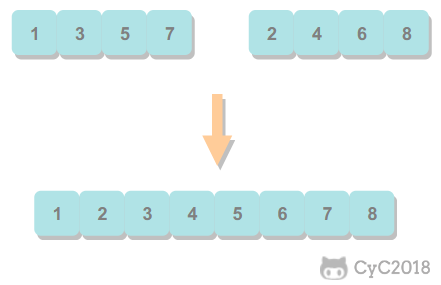## 1. 归并方法

``````public abstract class MergeSort<T extends Comparable<T>> extends Sort<T> {

protected T[] aux;

protected void merge(T[] nums, int l, int m, int h) {

int i = l, j = m + 1;

for (int k = l; k <= h; k++) {
aux[k] = nums[k]; // 将数据复制到辅助数组
}

for (int k = l; k <= h; k++) {
if (i > m) {
nums[k] = aux[j++];

} else if (j > h) {
nums[k] = aux[i++];

} else if (aux[i].compareTo(aux[j]) <= 0) {
nums[k] = aux[i++]; // 先进行这一步，保证稳定性

} else {
nums[k] = aux[j++];
}
}
}
}
``````

## 2. 自顶向下归并排序

``````public class Up2DownMergeSort<T extends Comparable<T>> extends MergeSort<T> {

@Override
public void sort(T[] nums) {
aux = (T[]) new Comparable[nums.length];
sort(nums, 0, nums.length - 1);
}

private void sort(T[] nums, int l, int h) {
if (h <= l) {
return;
}
int mid = l + (h - l) / 2;
sort(nums, l, mid);
sort(nums, mid + 1, h);
merge(nums, l, mid, h);
}
}
``````

## 3. 自底向上归并排序

``````public class Down2UpMergeSort<T extends Comparable<T>> extends MergeSort<T> {

@Override
public void sort(T[] nums) {

int N = nums.length;
aux = (T[]) new Comparable[N];

for (int sz = 1; sz < N; sz += sz) {
for (int lo = 0; lo < N - sz; lo += sz + sz) {
merge(nums, lo, lo + sz - 1, Math.min(lo + sz + sz - 1, N - 1));
}
}
}
}

``````

# 快速排序

## 1. 基本算法

• 归并排序将数组分为两个子数组分别排序，并将有序的子数组归并使得整个数组排序；
• 快速排序通过一个切分元素将数组分为两个子数组，左子数组小于等于切分元素，右子数组大于等于切分元素，将这两个子数组排序也就将整个数组排序了。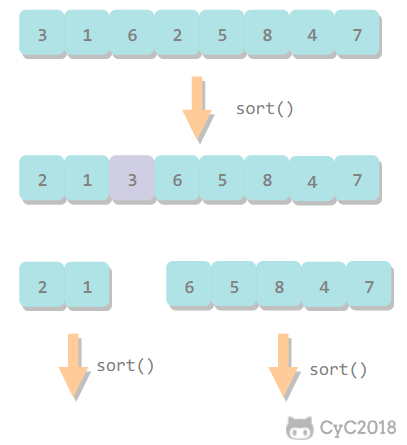``````public class QuickSort<T extends Comparable<T>> extends Sort<T> {

@Override
public void sort(T[] nums) {
shuffle(nums);
sort(nums, 0, nums.length - 1);
}

private void sort(T[] nums, int l, int h) {
if (h <= l)
return;
int j = partition(nums, l, h);
sort(nums, l, j - 1);
sort(nums, j + 1, h);
}

private void shuffle(T[] nums) {
List<Comparable> list = Arrays.asList(nums);
Collections.shuffle(list);
list.toArray(nums);
}
}
``````

## 2. 切分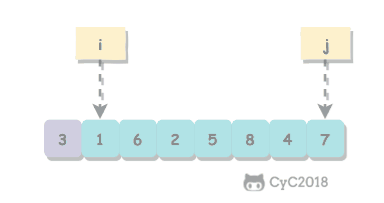``````private int partition(T[] nums, int l, int h) {
int i = l, j = h + 1;
T v = nums[l];
while (true) {
while (less(nums[++i], v) && i != h) ;
while (less(v, nums[--j]) && j != l) ;
if (i >= j)
break;
swap(nums, i, j);
}
swap(nums, l, j);
return j;
}
``````

## 4. 算法改进

#### 4.3 三向切分

``````public class ThreeWayQuickSort<T extends Comparable<T>> extends QuickSort<T> {

@Override
protected void sort(T[] nums, int l, int h) {
if (h <= l) {
return;
}
int lt = l, i = l + 1, gt = h;
T v = nums[l];
while (i <= gt) {
int cmp = nums[i].compareTo(v);
if (cmp < 0) {
swap(nums, lt++, i++);
} else if (cmp > 0) {
swap(nums, i, gt--);
} else {
i++;
}
}
sort(nums, l, lt - 1);
sort(nums, gt + 1, h);
}
}
``````

## 5. 基于切分的快速选择算法

``````public T select(T[] nums, int k) {
int l = 0, h = nums.length - 1;
while (h > l) {
int j = partition(nums, l, h);

if (j == k) {
return nums[k];

} else if (j > k) {
h = j - 1;

} else {
l = j + 1;
}
}
return nums[k];
}
``````

# 堆排序

## 1. 堆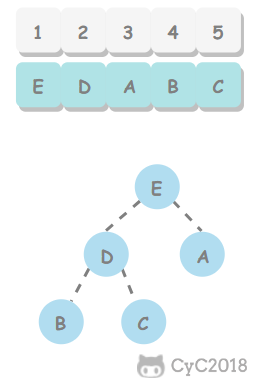``````public class Heap<T extends Comparable<T>> {

private T[] heap;
private int N = 0;

public Heap(int maxN) {
this.heap = (T[]) new Comparable[maxN + 1];
}

public boolean isEmpty() {
return N == 0;
}

public int size() {
return N;
}

private boolean less(int i, int j) {
return heap[i].compareTo(heap[j]) < 0;
}

private void swap(int i, int j) {
T t = heap[i];
heap[i] = heap[j];
heap[j] = t;
}
}
``````

## 2. 上浮和下沉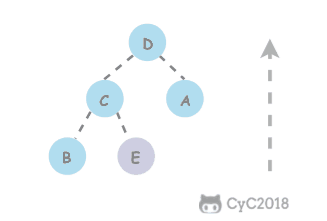``````private void swim(int k) {
while (k > 1 && less(k / 2, k)) {
swap(k / 2, k);
k = k / 2;
}
}
``````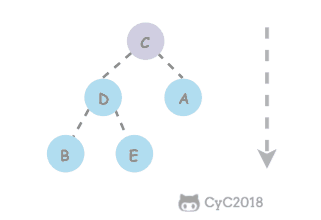``````private void sink(int k) {
while (2 * k <= N) {
int j = 2 * k;
if (j < N && less(j, j + 1))
j++;
if (!less(k, j))
break;
swap(k, j);
k = j;
}
}
``````

## 3. 插入元素

``````public void insert(Comparable v) {
heap[++N] = v;
swim(N);
}
``````

## 4. 删除最大元素

``````public T delMax() {
T max = heap;
swap(1, N--);
heap[N + 1] = null;
sink(1);
return max;
}
``````

## 5. 堆排序

#### 5.1 构建堆#### 5.2 交换堆顶元素与最后一个元素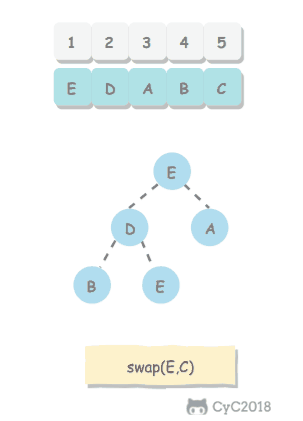``````public class HeapSort<T extends Comparable<T>> extends Sort<T> {
/**
* 数组第 0 个位置不能有元素
*/
@Override
public void sort(T[] nums) {
int N = nums.length - 1;
for (int k = N / 2; k >= 1; k--)
sink(nums, k, N);

while (N > 1) {
swap(nums, 1, N--);
sink(nums, 1, N);
}
}

private void sink(T[] nums, int k, int N) {
while (2 * k <= N) {
int j = 2 * k;
if (j < N && less(nums, j, j + 1))
j++;
if (!less(nums, k, j))
break;
swap(nums, k, j);
k = j;
}
}

private boolean less(T[] nums, int i, int j) {
return nums[i].compareTo(nums[j]) < 0;
}
}
``````

# 小结

## 2. Java 的排序算法实现

Java 主要排序方法为 java.util.Arrays.sort()，对于原始数据类型使用三向切分的快速排序，对于引用类型使用归并排序。